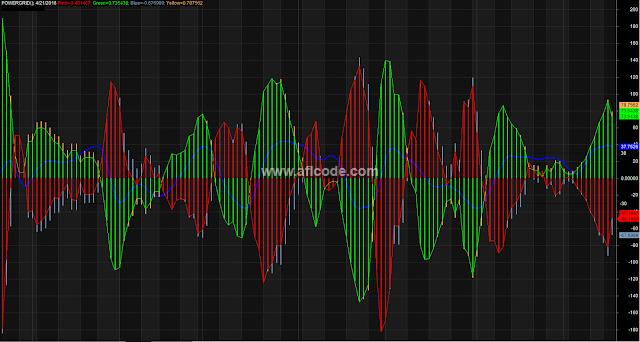Histogram Flag Trading Indicators

```//www.aflcode.com
/******************** Preliminaries *******************************/
PositionSize = 10000;
scale = Param("scale", 100, 100, 1000, 100);

/******************** Generate Histogram data *********************/
//= Red Bars ===================
Gr1 = MACD(22,6);
Gr2 = Signal(22,6,4);
Diffr = Gr1-Gr2;

//= Green Bars ===================
Gr1 = MACD(8, 14);
Gr2 = Signal(8, 14, 10);
Diffg = Gr1-Gr2;

//= Blue Bars ===================
Gr1 = MACD(22,11);
Gr2 = Signal(22,11,4);
Diffb = Gr1-Gr2;

//= Yellow Bars ===================
Gr1 = MACD(12,15);
Gr2 = Signal(12,15,8);
Diffy = Gr1-Gr2;

CR = (LastValue(Highest(diffr))/LastValue(Highest(diffr))) * Diffr;
CG = (LastValue(Highest(diffr))/LastValue(Highest(diffg))) * Diffg;
CB = (LastValue(Highest(diffr))/LastValue(Highest(diffb))) * Diffb;
CY = (LastValue(Highest(diffr))/LastValue(Highest(diffy))) * Diffy;

/***************** Generate Stochastics ***************************/

Stoch_K = StochK(17, 3);
Stoch_D = StochD(17, 3, 3);

/**************** Generate Bollinger Band "stuff" *****************/

BBT = BBandTop(C, 21, 2);
BBB = BBandBot(C, 21, 2);
BBML = (BBT + BBB) / 2;
QBB = (BBT-BBB) / 4;
BBUQ = BBML + QBB;
BBLQ = BBML - QBB;
CinTopQuarter = (C >= BBUQ); // 1 if C in upper quarter, 0 otherwise
CinBottomQuarter = (C <= BBLQ); // 1 if C in lower quarter, 0 otherwise

/**************** Generate Buy / Sell Signals *********************/

// 3 Options for initial Buy condition
// 1a) Buy when Green bar crosses from below to above zero line
// 1b) Buy as the Green (below zero) bar starts to turn up and the Red(above zero) starts to turn down
// 1c) Buy as the Green starts to turn up with Yellow showing belowthe Green AND the Red starts to turn down

Buy1 = Cross(CG , 0); // option 1a
//Buy1 = ((CG < 0) AND (CR > 0) AND (Ref(CG,-1) < CG) AND (Ref(CR,-1)> CR)); // option 1b
//Buy1 = (((CG < 0) AND (CR > 0) AND (Ref(CG,-1) < CG) AND (Ref(CR,-1)> CR) AND (CY < CG))); // option 1c

// 2) Stochastic has remained flat ....below the 20 line for severaldays AND then crosses the line
// Note: I don't know how to encode "flat",
// I use the STOCH D,
// "several days" means 3
// 3) The price is at the ... bottom of the Bollinger Band

Buy2 = (Ref(Stoch_D, -3) < 20) AND
(Ref(Stoch_D, -2) < 20) AND
(Ref(Stoch_D, -1) < 20) AND
Cross(Stoch_D, 20);

// 3 Options for intial Sell condition
// 1a) Sell when Green bar crosses from above to below zero line
// 1b) Sell as the Green starts to turn down and the Red starts to turn up
// 1c) Sell as the Green starts to turn down and the Red starts toturn up with Blue showing below the Red

Sell1 = Cross(0, CG); // option 1a
//Sell1 = ((CG > 0) AND (CR < 0) AND (Ref(CG, -1) > CG) AND(Ref(CR,-1) < CR)); // option 1b
//Sell1 = (((CG > 0) AND (CR < 0) AND (Ref(CG, -1) > CG) AND(Ref(CR,-1) < CR) AND (CB < CR))); // option 1c

// 2) Stochastic has remained flat above the 80.... line for severaldays AND then crosses the line
// Note: I don't know how to encode "flat",
// I use the STOCH D,
// "several days" means 3
// 3) The price is at the top ... of the Bollinger Band

Sell2 = (Ref(Stoch_D, -3) > 80) AND
(Ref(Stoch_D, -2) > 80) AND
(Ref(Stoch_D, -1) < 80) AND
Cross(80, Stoch_D);

Sell3 = CinTopQuarter;

Sell = Sell1 AND Sell3; // Sell2 is not used as it's too restrictive

// Remove extraneous Buy / Sell signals

/***************************** Indicator Plotting ***************/
_N(Title = Name() + "(" + FullName() + "); " + Date() +
" \\c32Red=" + CR + ";" + " \\c43Green=" + CG + "; \\c37Blue=" + CB
+ "; \\c33Yellow=" + CY +
"\n\\c16 4-MACD Exploration");

Plot (CR*scale, "", colorRed, 2+4);
Plot (CG*scale, "", colorBrightGreen, 2+4);
Plot (CB*scale, "", colorLightBlue, 2+4);
Plot (CY*scale, "", colorLightOrange, 2+4);
Plot (0, "", 16);
Plot (CR*scale, "", colorRed, 1);
Plot (CG*scale, "", colorBrightGreen, 1);
PlotShapes(IIf(Sell,shapeDownArrow,shapeNone),colorRed);

// Stoch_D is moved down by 50 points so that it's equilibrium line is is 0 rather than 50.
// The 20 and 80 Stochastic lines are now 30 and -30, respectively.
// This allows the stochastic to be plotted directly over the 4 MACD bars, which are centered
// around 0 as well.
Plot (Stoch_D-50, "", 29, 1+4+8);
Plot (30, "", 16);
Plot (-30,"", 16);

// Signals
SellSignals = Sell * shapeDownArrow;
PlotShapes (BuySignals, colorGreen, 0, Stoch_D-50, -20);
PlotShapes (Sellsignals, colorRed, 0, Stoch_D-50, -20);

/*************************** Exploration ***********************/
MinVolume = 1000000;
CloseTimesVolume = C * V;
MinCloseVolume = MA(CloseTimesVolume, 50) > MinVolume;
MinPrice = 5;
MaxPrice = 50;